Reach Us+44-1522-440391
A Maximum-Type Association Test for Censored Time-to-Event Data | OMICS International
Journal of Biometrics & Biostatistics

Like us on:

All submissions of the EM system will be redirected to Online Manuscript Submission System. Authors are requested to submit articles directly to Online Manuscript Submission System of respective journal.

# A Maximum-Type Association Test for Censored Time-to-Event Data

Esther Herberich1 and Ludwig A Hothorn2*

1Institute of Statistics, Ludwig-Maximilians-University Munich, Germany

2Institute of Biostatistics, Leibniz University Hannover, Germany

*Corresponding Author:
Ludwig A Hothorn
Institute of Biostatistics
Leibniz University Hannover, Germany
Tel: 49-511-762-5566
E-mail: [email protected]

Received Date: October 10, 2013; Accepted Date: November 18, 2013; Published Date: November 23, 2013

Citation: Herberich E, Hothorn LA (2013) A Maximum-Type Association Test for Censored Time-to-Event Data. J Biomet Biostat 4: 178. doi: 10.4172/2155-6180.1000178

Copyright: © 2013 Herberich E, et al. This is an open-access article distributed under the terms of the Creative Commons Attribution License, which permits unrestricted use, distribution, and reproduction in any medium, provided the original author and source are credited.

Visit for more related articles at Journal of Biometrics & Biostatistics

#### Abstract

Background: Testing the association between a diallelic marker and a censored time-to-event trait is a specific problem in population-based association studies. For a certain gene, the mode of inheritance may be of particular interest. Therefore, the principle of maximum-type tests (or minimum p procedure) is modified for continuous traits, especially for censored time-to-event data.
Results: We propose a Marcus-type multiple contrast test for a single censored time-to-event trait in a populationbased study assuming a Cox proportional hazard model. Using simulations we worked out the limitation of this asymptotic approach: sufficient sample sizes and non-rare alleles are required. A user-friendly implementation of this method is available in the survival and multcomp packages of the statistical software R.
Conclusions: The proposed approach can be used for the analysis of individual SNPs when censored time-to-event data in population-based association studies are of interest. The approach allows both a global claim of association and determination of the particular underlying mode of inheritance. The mode-specific hazard ratios and their lower simultaneous confidence limits provide information about statistical significance and genetic relevance.

#### Keywords

Max-test; Censored time-to-event trait; Populationbased association study

#### Introduction

Particular SNPs in population-based association studies can be analyzed using a maximum test, such as the MAX-3 test. This approach is widely used for proportions in the case control design and for continuous traits. Here, an extension is proposed for censored time-to-event traits using a Marcus-type multiple contrast test under the assumptions of the Cox proportional hazard model. Simulations revealed serious limitation of this asymptotic approach as sufficient sample sizes and non-rare alleles are required. Both a global claim of association and the particular underlying mode of inheritance can be identified. The mode-specific hazard ratios and their lower simultaneous confidence limits provide information about statistical significance and genetic relevance. A user-friendly implementation of this method is available in the survival and multcomp packages of the statistical software R.

Genome-wide association studies involving large population-based samples are used to identify common variants that affect a particular trait. Most of these studies compare the allele frequencies of di-allelic markers in cases and controls using the Cochran-Armitage trend test . Because the mode of inheritance at a given locus is often unknown, a maximum-test (minimum p approach, respectively) based on three mode-specific standardized Cochran Armitage trend tests have been proposed . Alternatively, continuous endpoints (i.e., quantitative traits), such as gene expression, are commonly analyzed using a linear regression model of genotype scores x=(0, 1, 2) adjusted for covariates . A special case of quantitative traits is time-to-event data with right censoring. For example, in a study of the survival of 116 female mice with the three genotypes aa, Aa and AA at the marker DM13D147 in chromosome 13 after an infection with Listeria monocytogenes , the raw data consist of the following three items (available in the R package qtl ): survival time (pheno), genotype group (geno) and censoring status (cens) (Table 1). The related Kaplan-Meier estimators reveal substantial differences in survival between the three genotype groups aa, Aa and AA, in which A is assumed to be the high risk allele:

pheno geno cens
1 118.32 AA TRUE
2 264 Aa FALSE
3 194.92 Aa TRUE
4 264 Aa FALSE
5 145.42 Aa TRUE
6 177.23 Aa TRUE
7 264 aa FALSE
8 76.67 AA TRUE
9 90.75 AA TRUE
10 76.17 Aa TRUE
. . . .
. . . .
. . . .
115 76.48 AA TRUE
116 116.47 Aa TRUE
117 116.52 Aa TRUE
118 139.55 Aa TRUE
119 264 Aa FALSE
120 116.2 Aa TRUE

Table 1: Raw data.

Figure 1 shows that the survival function of the heterozygous genotype, Aa, is not symmetrical to the functions of the two homozygous genotypes, aa and AA, which would be an indicator of an additive mode of inheritance. Instead, the heterozygous genotype is close to the non-risk homozygous, aa, indicating a recessive mode of inheritance. The idea of the maximum test is sensitivity to each of the basic modes of inheritance (i.e., additive, recessive, and dominant). Although reporting tiny p-values for the list of top-k SNPs is common nowadays, the ‘Strengthening the Reporting of Genetic Association studies’ report  recommended reporting appropriate effect size estimators and their confidence intervals. To compare survival functions, the hazard ratio is the appropriate effect size and simultaneous confidence intervals for a maximum-test for the three basic genetic models (i.e. additive, recessive, and dominant mode), are estimated. We propose a testing procedure that not only is sensitive to these three alternatives, but also able to determine which of the alternatives is likely using the diagnostic characteristics of simultaneous confidence intervals. We use the multiple contrast test approach , extended to the Cox proportional hazard model. This test is not likely applicable to genome-wide studies, merely because of the long computation time (about 0.03 sec on i7-4600 CPU per phenotype and SNP), but it can be used for specific analysis of the top-k SNPs or a priori genes of interest.

Figure 1: Kaplan-Meier curves displaying the Cumulative Survival Rate for the three genotype groups in female mice infected with Listeria monocytogenes .

Here, we describe an asymptotic multiple contrast test for a censored time-to-event trait assuming the Cox proportional hazards model based on the simultaneous inference approach in general parametric models . This is an extension of a related scores test for the generalized linear model  for censored time-to-event traits.

#### Methods

Marcus-type association test for censored time-to-event data

We consider three genotype groups i ∈ {aa, Aa, AA} with ni subjects carrying genotype i. A denotes the high risk allele and a denotes any other allele. To describe the effect of genotype i and, when applicable, the effects of other covariates on the hazard of death we use a Cox proportional hazard model: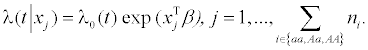The vector xj contains the covariates of the jth individual including the genotype i; the vector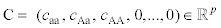with restriction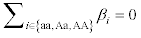includes the genotype effects and the effects of further covariates; λ0(t) denotes the baseline hazard rate at time t and is assumed to be identical for all individuals.

Letbe a vector of contrast coefficients fulfilling the constraint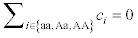For the sake of simplicity, we assume a model with the genotype as single covariate in the following, i.e., C=(caa, cAa, cAA) and β=(βaa, βAa, βAA). If the elements of vector c fulfill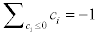and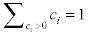the linear combination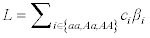can be interpreted as a difference of weighted averages of genotype effects.

We consider three genetic contrasts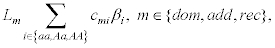each corresponding to one of the three genetic models, i.e. for each genetic model an individual statistic is computed. These contrasts are formulated by the so-called Marcus-type contrast matrix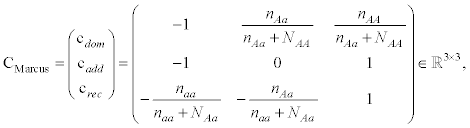(1)

whose elements cmi are the contrast coefficients. The product of each row vector of C and the vector of genotype effects β=(βaa, βAa, βAA) corresponds to one linear combination Lm, m ∈ {dom, add, rec} associated with a specific genetic model.

In case of a dominant mode of inheritance, the effects βAa and βAA on the hazard rate are identical. Therefore a genetic contrast for this mode can be expressed by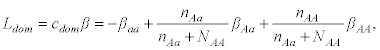which denotes the difference between the pooled effects of genotypes Aa and AA. Analogously, in case of a recessive mode of inheritance, the effects βaa and βAa on the hazard rate are identical. A recessive genetic contrast can be specified by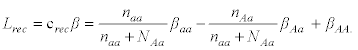The genetic contrast for an additive model can be expressed by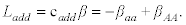Thus, the case of no global genetic effect is characterized by βaaAAA or, equivalently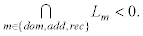We can test for a genetic model by performing a one-sided union-intersection test on the three linear combinations Lm with control of the FWER over all three contrasts. That is, we test the intersection of the elementary null hypotheses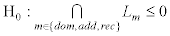versus the union of the elementary alternative hypotheses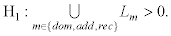This multiple contrast test was already described for normally distributed variables . Therefore, we denote this as the Marcus-type multiple contrast test.

Instead of multiple tests, lower simultaneous confidence intervals for the linear combinations Lm can be used. Exponentiating the confidence limits leads to confidence intervals for exp (Lm), which can be interpreted as a hazard ratio of the weighted average of the genotype effects. The presence of an association between genotype and trait is indicated if at least one of the three confidence intervals for the hazard ratios exp (Lm) excludes the value 1.

In other words, the above procedure tests the null hypothesis that no genetic effect exists against the three alternatives that the mode of inheritance is dominant, additive, or recessive.

An adjustment is needed to ensure that the overall hypothesis (no global genetic effect) is tested at level α. The three local hypotheses are positively correlated, and this correlation is included in the test procedure in order to prevent the overall test from being too conservative. By testing three different local hypotheses, the procedure is sensitive to three different genetic models and has greater power to detect an association when the mode of inheritance is not additive.

Approximate lower confidence limits for one contrast of genotype effects

In the Cox proportional hazards model, parameter estimates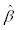are obtained by maximization of the partial likelihood . The maximum partial likelihood estimates are asymptotically normally distributed . The point estimator for a single linear combination L is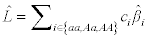and the lower (1-α) Wald confidence limit for L is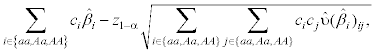where z1-α denotes the (1−α) quantile of the standard normal distribution and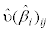the element in the ith row and jth column of the matrix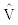.is the inverse of the observed Cox information matrix and used as an estimation of the covariance of.

A lower (1−α) Wald confidence limit for the hazard ratio exp (L) is given by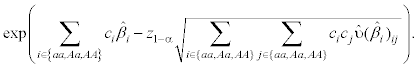In case of non-proportional hazards the accelerated failure time model can be used. In addition, the frailty Cox model can be used to model clustered survival data, such as when considering multiple studies in a meta-analysis. Both approaches are available for multiple contrast tests .

Approximate simultaneous lower confidence limits for multiple of genotype effects

According to Hothorn et al. , limits of approximate lower simultaneous confidence intervals for several linear combinations of model parameters Lm can be constructed by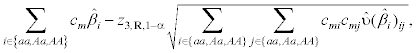where z3,R,1−α is the upper equicoordinate (1−α) quantile of the multivariate normal distribution with expectation 0 and correlation matrix R and Φ3 (q; 0, R) the associated cumulative density function. The quantile z3,R,1−α is chosen such that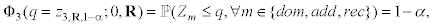where Zm is the mth element of a trivariate normal random vector Z ~ N (0, R). The probability that atleast one of the simultaneous confidence intervals does not include the true value of the associated contrast Lm is α with n→ ∞. Control of the FWER is achieved using quantiles that take the number of estimated contrasts and correlation between them into account.

Again, exponentiating the lower limit leads to simultaneous confidence intervals for multiple hazard ratios: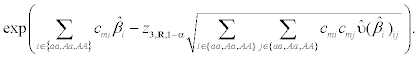#### Results

Simulations

To evaluate the performance of the proposed method we estimated the type I error rate and power using simulations in the open-source software R . Each simulation step was repeated 10,000 times.

The trait genotypes for N=500, 1000, 2000 subjects were randomly drawn from a multinomial distribution assuming Hardy-Weinberg equilibrium. Allele frequencies were chosen p=0.5 at trait locus, pm=0.05, 0.1, 0.3, 0.5 at trait marker, and linkage disequilibrium (LD) was chosen δ=0.025, 0.05, 0.1, 0.2. Phenotypic time-to-event data were generated according to Bender et al.  using a Weibull distribution with baseline hazard rate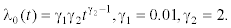Censoring times were generated from a uniform distribution on the interval [0,τ] with the τ chosen such that the censoring rate was approximately 20%.

The desired confidence level was 1−α=0.95.

For estimation of the probability of type I error, data were generated under the null hypothesis βaaAaAA=0, i.e. corresponding to a hazard ratio of 1. In one setting the model was investigated without additional covariates besides the genotype. In another setting, the model was investigated with two covariates: x1 uniformly distributed on [2,4] without an effect on the hazard rate, i.e. β1=0, and x2 uniformly distributed on [0,4] with effect β2=0.5. The family wise error rate (FWER), that is the probability of falsely detecting any mode of inheritance, was used as measure of the type I error and estimated by the proportion of datasets in which at least one simultaneous confidence interval for Marcus-type hazard ratios did not include the value 1.

For estimation of the power phenotypic values were simulated using genotype effects βaaAa=0 and βAA ∈ [0,2] for a recessive mode of inheritance, βaa=0, βAa [0,1] and βAA=2. βAa for an additive mode of inheritance, and βaa=0 and βAaAA ∈ [0,2] for a dominant mode of inheritance. These genotype-specific effects correspond to mode of inheritance-specific hazard ratios HR ∈ [1,4,7]. Each value of power was estimated by the proportion of datasets in which the correct mode of inheritance was detected by the simultaneous lower confidence intervals.

The estimated type I error (FWER) is shown in Figure 2. For fixed sample size, the procedures get more liberal with increasing disbalancy of allele frequencies and/or lower LD. For settings with rather balanced allele frequencies, i.e. pm=0.3, 0.5, a sample size of N=500 is sufficient to ensures FWER control even when LD is low. For settings with unbalanced allele frequencies, i.e. pm=0.05, 0.1 larger samples are required. A sample size of N=2000 for pm=0.1, and a sample size of N=5000 for pm=0.05 provides FWER control (results for N=5000 not shown). In the setting with covariates, the FWER is slightly higher than in the model with the genotype as single covariate.

Figure 2: Estimated FWER in the setting without covariates besides’ the genotype (upper row) and in the setting with further covariates (lower row).

The power of the procedure to identify the correct genetic model is given in Figure 3 for sample sizes of N=1000. The power increases with higher linkage disequilibrium in all models. The power is considerably higher for the dominant and additive mode of inheritance compared to the recessive mode of inheritance, with the latter showing very poor power except when allele frequency was pm=0.5 at trait marker. In the dominant model, the power decreases with increasing frequencies of the rare allele, whereas the power increases with increasing frequency of the rare allele in the recessive model. The power in the additive model is similar for all allele frequencies. With increasing sample size (N=500 vs. N=1000 vs. N=2000), no general increase in the power to detect the correct mode of inheritance can be found (Power curves for N=500 and N=2000 not shown.). In some settings the mode-specific power increases slightly, whereas in some settings the mode-specific power increases. The power to detect any association increases with increasing sample size, but more often incorrect mode of inheritance is chosen. When the high-risk allele is rare and thus genotype frequencies are unbalanced, an additive mode of inheritance is more often stated to be dominant, and a recessive mode of inheritance is more often stated to be additive (Figure 3).

Figure 4 shows the power of the procedure to detect any association between genotype and trait. The extent to which the procedure’s power to detect the correct mode of inheritance and the power to detect any association differ depends on the underlying mode of inheritance and the disbalancy of allele frequencies. A ’symmetrical’ relation of the modes of inheritance and the differences between overall and modespecific power exists, which is caused by the fact that the three modes of inheritance are ’symmetrical’ for the alleles. When high-risk alleles are rare (pm=0.05, 0.1), the difference in overall power and mode-specific power is negotiable for the dominant, considerable for the recessive, and intermediate for the additive mode of inheritance. When highrisk alleles are frequent (pm>0.75) the difference in overall power and mode-specific power is negotiable for the recessive, considerable for the dominant, and again intermediate for the additive mode of inheritance. When alleles frequencies are balanced, the difference in overall power and mode-specific power is negotiable for the additive model, and intermediate for the dominant and recessive modes of inheritance (Figure 4).

Evaluation of the example

The Listeria example described above was analyzed using the new Marcus-type association test for censored time-to-event data. Simultaneous lower 95% confidence intervals for contrasts of genotype effects corresponding to the three genetic models were computed using the R  packages survival  and multcomp . The estimated hazard ratios and simultaneous lower confidence limits for the three basic modes of inheritance are given in Table 2.

Inheritance-specific contrast Hazard ratio Lower confidence limit
Cdom 1.56 0.82
Cadd 3.16 1.60
Crec 3.50 2.23

Table 2: Estimated inheritance-specific hazard ratios and their simultaneous lower confidence limit for marker DM13D147 at chromosome 13.

Clearly, the largest hazard ratio with the most distant lower confidence limit was determined for the recessive mode of inheritance (abbreviated with Crec). Mice homozygous for the high risk allele had a greater chance of a short survival time compared to animals carrying either one or two non-risk alleles (genotypes Aa and aa). With a probability of 95% animals carrying two high risk alleles had at least a 2.23-fold greater hazard.

Software

The simultaneous confidence intervals for hazard ratios obtained from a Cox proportional hazard model can be computed using the coxph function in the package survival . The lower simultaneous confidence intervals for Marcus-type hazard ratios can be computed by the function glht in the package multcomp [17,18]. Intervals for the hazard ratios were obtained by exponentiating the estimated confidence limits. Alternatively, adjusted p-values can be calculated for the corresponding multiple tests. The package multcomp employs the algorithms used for computing the multivariate normal quantiles  implemented in the package mvtnorm .

#### Conclusions

Evaluation of selected SNPs by the proposed Marcus-type multiple contrast test for censored time-to-event data in population-based association studies is useful in several aspects. First, the most likely underlying mode of inheritance, not just a global association, can be concluded. The outcomes, i.e., the mode-specific hazard ratios and their lower simultaneous confidence limits, allow interpretation in terms of both statistical significance and medical relevance. This asymptotic approach is limited to study designs with sufficient sample sizes, such as 1000 or more, and is limited to non-rare alleles. Real data can be analyzed easily using the R packages survival and multcomp. Straight forward extensions for data with non-proportional hazards and/or multiple studies (i.e., meta-analysis) are possible.

#### Acknowledgements

This work was supported in part by HO 1687/9-1 for the second author (LAH).

#### References

Select your language of interest to view the total content in your interested language

### Article Usage

• Total views: 12234
• [From(publication date):
December-2013 - Aug 25, 2019]
• Breakdown by view type
• HTML page views : 8408
• PDF downloads : 3826

## Post your commentCan't read the image? click here to refresh
###### Peer Reviewed Journals

Make the best use of Scientific Research and information from our 700 + peer reviewed, Open Access Journals

International Conferences 2019-20

Meet Inspiring Speakers and Experts at our 3000+ Global Annual Meetings

Top